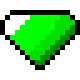# Pseudo 2D Convex Hull Generation

posted in: Programming |

While working with the separating axis theorem in a 2D XNA project, I started looking at ways to generate a tight-fitting convex hull around a sprite in order to implement more accurate collision detection than I would be able to with a standard bounding box.

There’s a whole slew of 2D convex hull generation algorithms out there — each of which could work with sprites by using any non-transparent pixels as a point set — and I hope to implement one in the future, but for the time being I’m using a simpler (albeit less robust) system that works well enough.

It works like so: rather than test every pixel, I use a select few which I’ve manually added prior to compilation with a color of RGB(254, 0, 254). Once retrieved, I sort them in a clockwise order about the sprite’s center and use them as vertices for a bounding polygon which may or may not be convex.Whether or not the polygon is convex obviously depends on how the original pixels were placed, so this isn’t a fool-proof system by any means. Assuming the input is good, though, the results are quite useful. Chuck in a simple pixel shader to remove the magenta pixels before rendering and it’s good to go.

The following method encapsulates all of the necessary steps, and it’s pretty heavily commented so it should make sense:

```public static Vector2[] GenerateHullFromTexture(Texture2D texture)
{
// Calculate center of texture
Vector2 texCenter = new Vector2(texture.Height / 2, texture.Width / 2);

// Dynamic List to hold each vertices specified in screen/pixel space
List<Vector2> vertexList = new List<Vector2>();

// Store texture's pixels in a 1D array
Color[] pixels = new Color[texture.Width * texture.Height];
texture.GetData(pixels);

// Loop through pixels and store any magenta ones as vertices
for (int x = 0; x < texture.Width; x++)
{
for (int y = 0; y < texture.Height; y++)
{
// The 2D cooridnate of each pixel in screen/pixel space
Color pixelXY = pixels[x + y * texture.Width];

// If the pixel is magenta, add its coordinate to the vertexList
if (pixelXY.R == 254 && pixelXY.G == 0 && pixelXY.B == 254)
}
}

// Convert the List of vertices to an array
Vector2[] vertexArray = vertexList.ToArray();

// Store angles between texture's center and vertex position vector
float[] angles = new float[vertexArray.Length];

// Calculate the angle between each vertex and the texture's /center
for (int i = 0; i < vertexArray.Length; i++)
{
angles[i] = AngleBetween2DVectors(vertexArray[i], texCenter);
vertexArray[i] -= texCenter;   // Offset vertex about texture center
}

// Sort angles into ascending order, use to put vertices in clockwise order
Array.Sort(angles, vertexArray);

return vertexArray; // Return the ordered vertex array
}
```

Where the AngleBetween2DVectors method is simply the following:

```public static float AngleBetweenVectors(Vector2 a, Vector2 b)
{
float angle = (float)Math.Atan2((double)(a.Y - b.Y),
(double)(a.X - b.X));
return angle;
}
```

I’m doing all this in the main Game class at the moment, but I’ll probably port the code over to a custom texture content processor at some point. I’d be happy to upload the source for that when it’s done if anyone’s interested (a long shot given that very few people know this blog exists!)

The following video gives an idea of what can be achieved by combing this functionality with the separating axis theorem (which should be the subject of my next post) for collision detection (click through to YouTube for a higher quality version):

### 3 Responses

1.##### Graeme Pollard

I know this is really old Karn, but I remember you posting on one of my blogs yonks ago, so thought I’d post. Anyway, it’s pretty easy to check if the points are actually convex. By definition, all of the inner angles of a convex shape are less than 180 degrees. And that’s really easy to check for each point by getting the angle between the 2 vectors between the point and the point either side of it. If one of them fails this convex criteria you can just ignore it. Anyway, cool stuff.

•##### Karn Bianco
2.